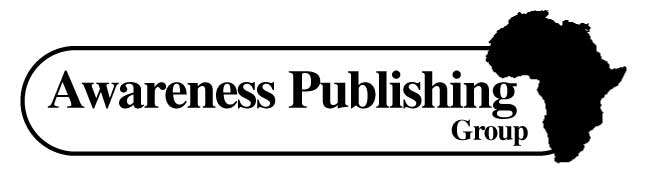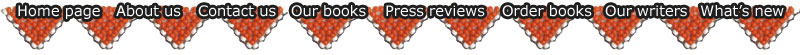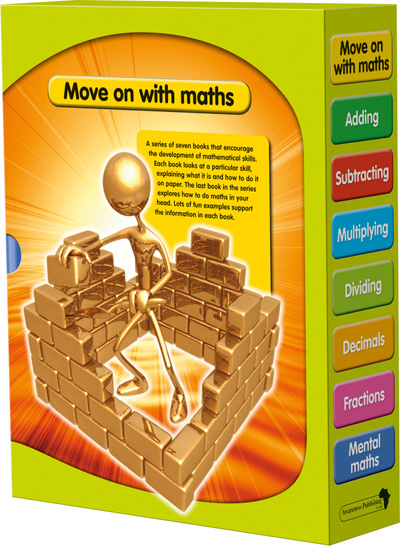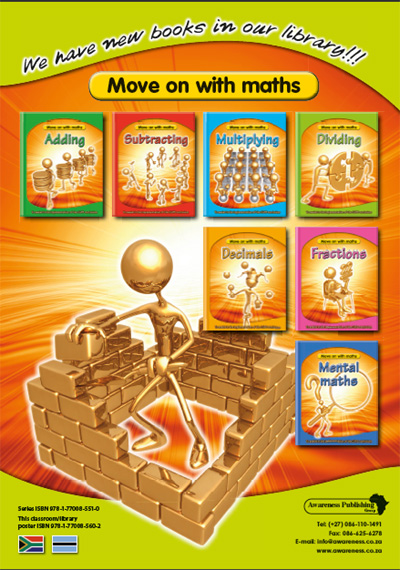>> Download the latest Awareness Publishing Book Catalogue
 Move on with maths series Set of 7 books 40pp each [Series ISBN 978-1-77008-551-0] | Adding | Subtracting | Multiplying | | Dividing | Decimals | Fractions | Mental maths | A series of seven books that encourage the development of mathematical skills. Each book looks at a particular skill, explaining what it is and how to do it on paper. The last book in the series explores how to do maths in your head. Lots of fun examples support the information in each book. EnglishAdding ISBN 978-1-77008-552-7 The book Adding covers counting; using rulers, patterns and columns; counting squares; and adding large numbers. It also discusses adding decimals, minus numbers and fractions. Lots of fun examples support the information in this book. This book contains a What symbols mean section and an Index. Brian Knapp and Colin Bass Contents Counting on Using a ruler to add Using two rulers to add Adding using patterns First adding facts Using columns to add Add in any order Starting an adding square Adding facts for 10 or more The complete adding square Adding from 10 to 99 Adding several numbers Adding bigger numbers Decimal numbers Adding minus numbers Adding fractions Adding to an equation Distance charts Subtracting ISBN 978-1-77008-553-4 The book Subtracting covers counting back; using rulers, shapes and columns; and subtracting large numbers. It explains the two ways of subtracting, which are exchanging and regrouping. The book also discusses subtracting large numbers, numbers with zeros, and decimals. This book contains a What symbols mean section and an Index. Brian Knapp and Colin Bass Contents Counting back A ruler for subtracting Counting on to find the difference Subtracting rulers Subtracting facts Pairs of subtracting facts Subtracting using shapes Subtracting in columns Subtracting 10 to 99 Subtracting by exchanging Subtracting by regrouping Subtracting large numbers Subtracting numbers with zeros Subtracting decimal numbers Subtracting in your head Subtracting and minus numbers Subtracting across zero Solving equations Multiplying ISBN 978-1-77008-554-1 The book Multiplying covers why we multiply, and how to do it. This includes multiplications squares and tables, short multiplication, and long multiplication using columns and grids. The book also discusses prime numbers and multiplying with a decimal. Lots of fun examples support the information in this book. This book contains a What symbols mean section and an Index. Brian Knapp and Colin Bass Contents Why multiply? How to multiply Multiply in any order Factors, multiples and products Starting a multiplication square Completing the square Multiplication tables Short multiplication More short multiplication Primes Square numbers and square roots Multiplying and adding Long multiplication by columns Long multiplying by grids Large numbers using columns Large numbers using grids Multiplying decimal numbers Finding an unknown amount Dividing ISBN 978-1-77008-555-8 The book Dividing covers why we divide, and how to do it. This includes short and long division, remainders, dividing with decimals, and working out the value for money. The book also discusses how fractions involve division, and how the words per and in can also mean divide. Lots of fun examples support the information in this book. This book contains a What symbols mean section and an Index. Brian Knapp and Colin Bass Contents Why divide? Sharing How dividing works Short division More short division Division and multiplication Long division Quick division tips Remainders Remainders in long division Division with decimal numbers Using remainders Value for money Fractional parts Fractions with the same value Per is a word for divide In can mean divide Solving division equations Decimals ISBN 978-1-77008-556-5 The book Decimals covers using the decimal comma; decimals in shapes, money and science; and adding, subtracting, multiplying and dividing decimals. The book also discusses recurring decimals, and rounding decimals up or down. Lots of fun examples support the information in this book. This book contains a What symbols mean section and an Index. Brian Knapp and Colin Bass Contents Using a decimal comma Decimals as shapes Decimals in money Decimals in science Adding with decimals Different decimal lengths Subtract decimals with the exchanging method Subtract decimals by regrouping Multiplying a decimal Multiplying with decimals Checking the decimal comma Dividing by a whole number Fractions to decimals Recurring decimals Rounding decimals Decimals to fractions Dividing decimals Comparing decimals Fractions ISBN 978-1-77008-557-2 The book Fractions covers adding and multiplying fractions, smaller fractions, fractions that have the same value and fractions that look different, as well as adding and subtracting fractions and mixed numbers. The book also discusses ratio and proportion, and percentages as fractions. Lots of fun examples support the information in this book. This book contains a What symbols mean section and an Index. Brian Knapp and Colin Bass Contents Finding out about fractions Quarters of many shapes Adding similar fractions Multiplying fractions Smaller and smaller fractions Mixing and matching Fractions with the same value Fractions that look different Adding unlike fractions Subtracting fractions Fractions bigger than one Adding mixed numbers Subtracting mixed numbers Ratio and proportion More on proportion Per cent Percentages as fractions Per cent more Mental maths ISBN 9978-1-77008-558-9 The book Mental maths covers doing mathematics in your head. This includes using pictures, splitting up numbers, working with odd and even numbers, and ways to add and subtract. The book also discusses using doubles, trebles and near equals. Lots of fun examples support the information in this book. This book contains a What symbols mean section and an Index. Brian Knapp and Colin Bass Contents Using pictures Splitting numbers up Put the big numbers first Choosing easy pairs Work with even numbers first Multiply odd by even numbers Sizani at the market When adding is better than multiplying Many ways to add Several ways to subtract Subtracting by adding on Subtracting across 100 Ziggie and Mtoto in Ghana Can’t remember your tables? Using doubles and trebles Near doubles More near equals The Molobis’ word game